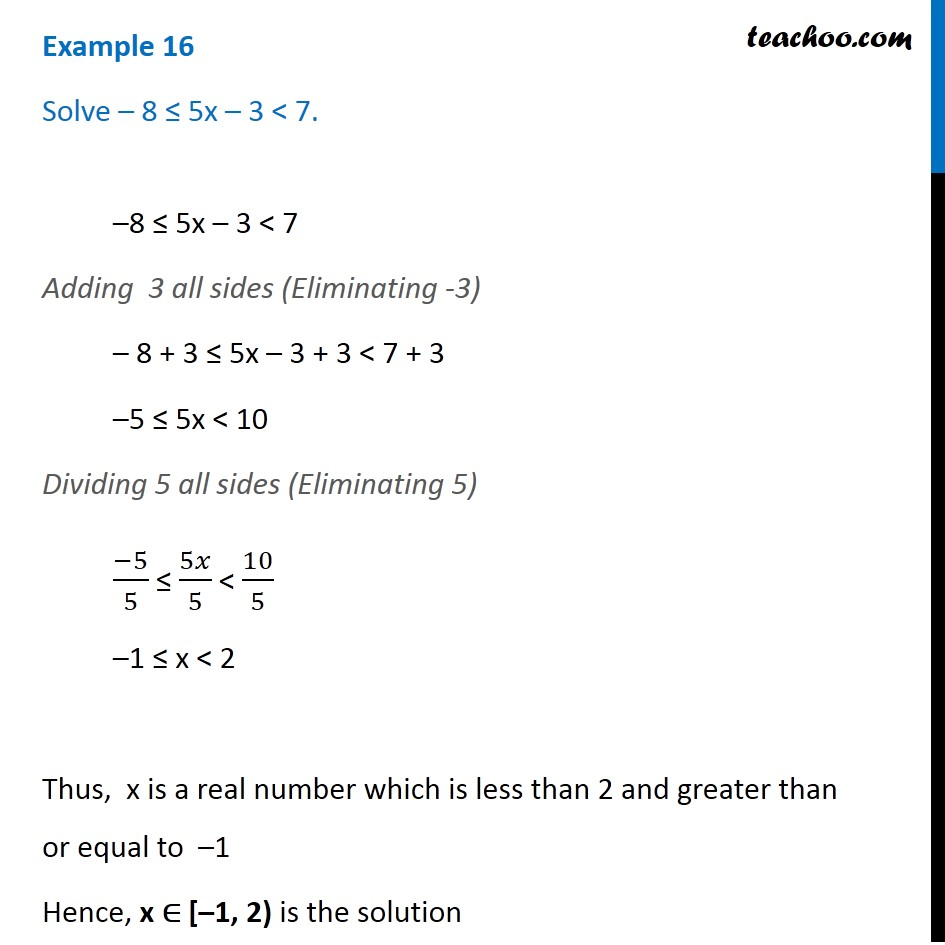Examples

Chapter 5 Class 11 Linear Inequalities
Serial order wiseLearn in your speed, with individual attention - Teachoo Maths 1-on-1 Class

### Transcript

Example 9 Solve – 8 ≤ 5x – 3 < 7. –8 ≤ 5x – 3 < 7 Adding 3 all sides (Eliminating -3) – 8 + 3 ≤ 5x – 3 + 3 < 7 + 3 –5 ≤ 5x < 10 Dividing 5 all sides (Eliminating 5) (−5)/5 ≤ 5𝑥/5 < 10/5 –1 ≤ x < 2 Thus, x is a real number which is less than 2 and greater than or equal to –1 Hence, x ∈ [–1, 2) is the solution